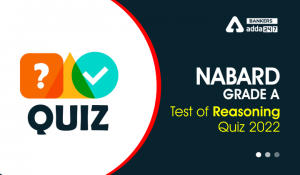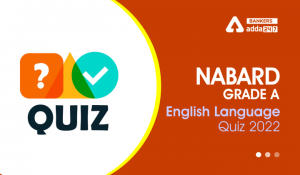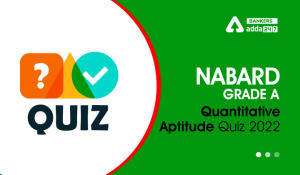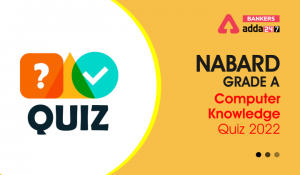Latest Banking jobs   »

# Reasoning Ability Quiz For NABARD Grade A 2022- 1st August

Directions (1-5): In these questions, relationship between different elements is show in the statements. The statements are followed by conclusions. Study the conclusions based on the given statements and select the appropriate answer:

(a) If only conclusion I follows.
(b) If only conclusion II follows.
(c) If either conclusion I or II follows
(d) If neither conclusion I nor II follows.
(e) If both conclusions I and II follow.

Q1. Statements: W≥Q>O=P>X=V<M=J
Conclusion I: V<W
II: V=W

Q2. Statements: Q>J≥L=C<B=E≥M>S=D
Conclusion I: L≥S
II: S<L

Q3. Statements: R≥E<V≤B≤N<S<O>Y>X
Conclusion I: S>E
II: O>R

Q4. Statements: U>R=S, V>W=F, S≤T≥V
Conclusion I: R≤V
II: R>V

Q5. Statements: T<J=K>L, N>M>R, R=T
Conclusion I: K>R
II: M>T

Direction (6-10): In these questions, relationships between elements is shown in the statements. These statements are followed by two conclusions. Give answer

(a) If only conclusion I follows.
(b) If only conclusion II follows.
(c) If either conclusion I or conclusion II follows.
(d) If neither conclusion I nor conclusion II follows.
(e) If both conclusions I and II follow.

Q6. Statements: G≤F=L≤J; J≤K=H
Conclusions: I. H=G
II. G<H

Q7. Statements: P<R<S<T>U
Conclusions: I. U<R
II. T>P

Q8. Statements: T>U≥V≥W; X<Y=W>Z
Conclusions: I. Z>U
II. W<T

Q9. Statements: K<L<M<N; M<O<P
Conclusions: I. P>K
II. N>O

Q10. Statements: B<A<C; A>D≤E
Conclusions: I. B≤E
II. C>E

Directions (11-15): In the following questions, the symbols #, %, \$, @ and © are used with the following meaning as illustrated below.
‘P # Q’ means ‘P is not smaller than Q’.
‘P % Q’ means ‘P is neither smaller than nor equal to Q’.
‘P \$ Q’ means ‘P is neither greater than nor equal to Q’.
‘P @ Q’ means ‘P is neither greater than nor smaller than Q’.
‘P © Q’ means ‘P is not greater than Q’.
Now, in each of the following questions assuming the given statements to be true, find which of the three Conclusions I, II and III given below them is/are definitely true and give your answer accordingly.

Conclusions: I. R%U
II. T#U
III. M%K
(a) Only I and III is true
(b) Only II and I is true
(c) Only II and III is true
(d) Only I is true
(e) None is true

Q12. Statements: D©G@ H, A%E#H, B\$D
Conclusions: I. B%A
II. E#D
III. G%E
(a) Only III and II is true
(b) Only II
(c) Only III is true
(d) Either I or III true
(e) None of these

Conclusions: I.Z%B
II. H@Z
III. G#A
(a) Only I is true
(b) I, II and III are true
(c) Either I or II true
(d) Only II is true
(e) None of these

Conclusions: I. L#Q
II. R%P
III. W%L
(a) Only II and III is true
(b) Only I is true
(c) Either I or III true
(d) Only II
(e) None of these

Q15. Statements: K%M@R, R#T%X, O\$X
Conclusions: I. M#T
II. R\$O
III. M%O
(a) Only I is true
(b) Only I and III is true
(c) Only II is true
(d) All are true
(e) None of these

Solutions

S1. Ans.(a)
Sol. I: V<W(true)
II: V=W(False)

S2. Ans.(d)
Sol. I: L≥S (False)
II: S<L (False)

S3. Ans.(a)
Sol. I: S>E (True)
II: O>R (False)

S4. Ans.(c)
Sol. I: R≤V (False)
II: R>V (false)

S5. Ans.(e)
Sol. I: K>R (True)
II: M>T(True)

S6. Ans. (c)
Sol. I. I.H=G (False)
II. G<H(False)

S7. Ans. (b)
Sol. I. U<R (False)
II.T>P(True)

S8. Ans. (b)
Sol. I. Z>U (False)
II.W<T(True)

S9. Ans. (a)
Sol. I. P>K (True)
II. N>O(False)

S10. Ans. (d)
Sol. I. B≤E (False)
II.C>E(False)

S11. Ans.(d)
Sol. I. R%U(true)
II. T#U(false)
III. M%K(false)

S12. Ans.(b)
Sol. I. B%A(false)
II. E#D(true)
III. G%E(false)

S13. Ans.(a)
Sol. I.Z%B(true)
II. H@Z(false)
III. G#A(false)

S14. Ans.(c)
Sol. I. L#Q(false)
II. R%P(false)
III. W%L(false)

S15. Ans.(b)
Sol. I. M#T(True)
II.R\$O(false)
III. M%O(True)#### Congratulations!Incorrect details? Fill the form again here

•Reasoning Ability Quiz For NABARD Grade ...
•English Quizzes For NABARD Grade A 2022-...
•Quantitative Aptitude Quiz For NABARD Gr...
•Computer Quiz For NABARD Grade A 2022- 1...
•Reasoning Ability Quiz For NABARD Grade ...
•English Quizzes For NABARD Grade A 2022-...